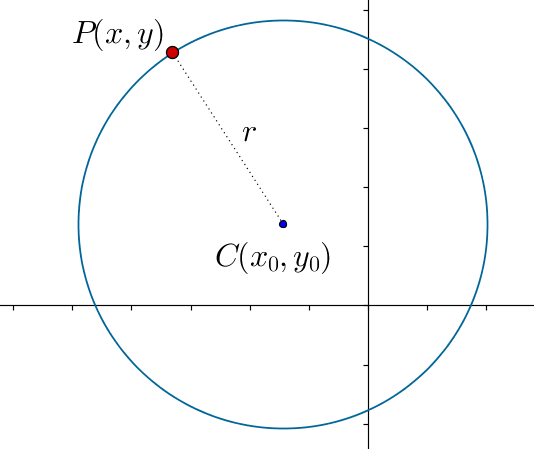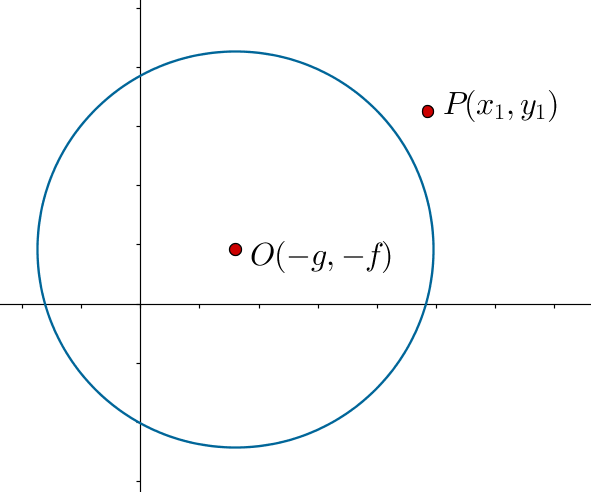# General Equation of a Circle

Go back to  'Coordinate-Geometry'

Let us analyze in more detail the equation of a circle of radius r centered at the point $$C\left( {{x_0},{y_0}} \right)$$ :The equation of this circle is

${\left( {x - {x_0}} \right)^2} + {\left( {y - {y_0}} \right)^2} = {r^2}$

If we square and expand, we have the following:

\begin{align}&{x^2} + {x_0}^2 - 2x{x_0} + {y^2} + {y_0}^2 - 2y{y_0} = {r^2}\\&\Rightarrow \,\,\,{x^2} + {y^2} - 2{x_0}x - 2{y_0}y + {x_0}^2 + {y_0}^2 - {r^2} = 0\end{align}

We see that there is an $${x^2}$$ term, a $${y^2}$$ term, a term which is linear in x, a term which is linear in y, and a constant term. Taking cue from this, we can write the general equation of a circle as follows:

$\underbrace {{x^2} + {y^2}}_{\scriptstyle{\rm{Square \,\,terms}}\atop{\scriptstyle{\rm{with \,\,equal}}\atop\scriptstyle{\rm{coefficients}}}} + \underbrace {2gx + 2fy}_{{\rm{Linear terms}}} + \underbrace c_{\scriptstyle{\rm{Constant}}\atop\scriptstyle{\rm{term}}} = 0$

Why have we taken the coefficients of x and y as 2g and 2f, rather than, say, g and f? These and other aspects of this general equation will become clearer when you delve deeper into this analysis at a later stage.

To summarize:

• The equation of a circle with center $$\left( {{x_0},{y_0}} \right)$$ and radius r can be written as

${\left( {x - {x_0}} \right)^2} + {\left( {y - {y_0}} \right)^2} = {r^2}$

• The general equation of a circle can be written as

${x^2} + {y^2} + 2gx + 2fy + c = 0$

If we rearrange the first equation into the general form, we have:

$\begin{array}{l}{x^2} + {y^2} + \left( { - 2{x_0}} \right)x + \left( { - 2{y_0}} \right)y\\ \,\,\,\,\,\;\;\;\;\;\;\;\;\;\; + \left( {{x_0}^2 + {y_0}^2 - {r^2}} \right) = 0\end{array}$

If we compare this with the general form a circle’s equation, we have:

\begin{align}& - 2{x_0} = 2g,\,\,\, - 2{y_0} = 2f,\,\,\,\left( {{x_0}^2 + {y_0}^2 - {r^2}} \right) = c\\&\Rightarrow \,\,\,{x_0} = - g,\,\,\,{y_0} = - f,\,\,\,r = \sqrt {{x_0}^2 + {y_0}^2 - c} \\&\qquad\qquad\qquad\qquad\qquad\quad\;\;= \sqrt {{g^2} + {f^2} - c} \end{align}

Thus, given the general form of a circle’s equation, which is

${x^2} + {y^2} + 2gx + 2fy + c = 0,$

we can say that the center of this circle is

$\left( {{x_0},{y_0}} \right) = \left( { - g, - f} \right),$

$r = \sqrt {{g^2} + {f^2} - c}$

Example 1: A circle is centered at $$\left( {3, - 2} \right)$$ and has a radius of 4 units. Write its equation in the general form.

Solution: We write the equation of the circle using its center and radius, and then rearrange to the general form:

\begin{align}&{\left( {x - 3} \right)^2} + {\left( {y - \left( { - 2} \right)} \right)^2} = {4^2}\\&\Rightarrow \,\,\,{\left( {x - 3} \right)^2} + {\left( {y + 2} \right)^2} = 16\\&\Rightarrow \,\,\,{x^2} - 6x + 9 + {y^2} + 4y + 4 = 16\\&\Rightarrow \,\,\,{x^2} + {y^2} - 6x + 4y - 3 = 0\end{align}

Example 2: Find the center and radius of the circle whose equation is $${x^2} + {y^2} - 4x - 6y - 12 = 0$$ .

Solution: Comparing the given equation to the standard equation $${x^2} + {y^2} + 2gx + 2fy + c = 0$$ , we note that

\begin{align}&2g = - 4,\,\,\,2f = - 6,\,\,\,c = - 12\\&\Rightarrow \,\,\,g = - 2,\,\,\,f = - 3,\,\,\,c = - 12\end{align}

Thus, the center of the circle is

$\left( { - g, - f} \right) = \left( {2,3} \right),$

$\begin{array}{l}r = \sqrt {{g^2} + {f^2} - c} \\\,\,\, = \sqrt {{{\left( { - 2} \right)}^2} + {{\left( { - 3} \right)}^2} - \left( { - 12} \right)} \\\,\,\, = \sqrt {25} = 5\end{array}$

Example 3: (a) The equation of a circle is

${x^2} + {y^2} + 2gx + 2fy + c = 0$

$$P\left( {{x_1},{y_1}} \right)$$ is a point not lying on this circle. What is the distance of P from the circle’s center?

(b) Find the condition so that a point $$P\left( {{x_1},{y_1}} \right)$$ lies outside the circle whose equation is

${x^2} + {y^2} + 2gx + 2fy + c = 0$

How will this condition change if P lies inside the circle?

Solution: Observe the following figure, which shows a circle with center O and a point P not lying on the circle:The distance OP can be calculated using the distance formula:

$\begin{array}{l}OP = \sqrt {{{\left( {{x_1} - \left( { - g} \right)} \right)}^2} + {{\left( {{y_1} - \left( { - f} \right)} \right)}^2}} \\\,\,\,\,\,\,\,\, = \sqrt {{x_1}^2 + {y_1}^2 + 2g{x_1} + 2f{y_1} + {g^2} + {f^2}} \end{array}$

(b) For P to lie outside the circle, the distance OP must be greater than the radius of the circle, which is $$r = \sqrt {{g^2} + {f^2} - c}$$. Thus, we have:

$\begin{array}{l}OP > r\\ \Rightarrow \,\,\,\sqrt {{x_1}^2 + {y_1}^2 + 2g{x_1} + 2f{y_1} + {g^2} + {f^2}}\\\;\;\;\;\;\;\;\;\;\;\;\;\;\;\;\;\;\; > \sqrt {{g^2} + {f^2} - c} \end{array}$

Squaring both sides, we have:

$\begin{array}{l}{x_1}^2 + {y_1}^2 + 2g{x_1} + 2f{y_1} + {g^2} + {f^2}\\\;\;\;\;\;\;\;\;\;\;\;\;\;\;\;\; > {g^2} + {f^2} - c\\ \Rightarrow \,\,\,{x_1}^2 + {y_1}^2 + 2g{x_1} + 2f{y_1} + c > 0\end{array}$

Let us denote the circle’s equation

${x^2} + {y^2} + 2gx + 2fy + c = 0$

by $$S\left( {x,y} \right) = 0$$ . Thus, for a point $$P\left( {{x_1},{y_1}} \right)$$ to lie outside the circle $$S\left( {x,y} \right) = 0$$, the required condition is

$S\left( {{x_1},{y_1}} \right) > 0$

Similarly, for $$P\left( {{x_1},{y_1}} \right)$$ to lie inside $$S\left( {x,y} \right) = 0$$ , the required condition is

$S\left( {{x_1},{y_1}} \right) < 0$

Of course, if $$P\left( {{x_1},{y_1}} \right)$$ on the circle, then $$S\left( {{x_1},{y_1}} \right) = 0$$, as the coordinates of P satisfy the circle’s equation.

Example 4: The equation of a circle is

${x^2} + {y^2} + 2x + 4y - 11 = 0$

Where do the points $$P\left( {2,4} \right)$$ and $$Q\left( {0,0} \right)$$ lie in relation to this circle?

Solution: Represent the equation of the circle by $$S\left( {x,y} \right) = 0$$. We substitute the coordinates of P and Q in the expression $$S\left( {x,y} \right)$$:

\begin{align}&S\left( {2,4} \right)= {2^2} + {4^2} + 2\left( 2 \right) + 4\left( 4 \right) - 11\\&\qquad\quad= 4 + 16 + 4 + 16 - 11 = 29 > 0\\&S\left( {0,0} \right) = {0^2} + {0^2} + 2\left( 0 \right) + 4\left( 0 \right) - 11\\&\qquad\quad= - 11 < 0\end{align}

Clearly, P lies outside the circle, while Q lies inside it.

Coordinate Geometry
Grade 9 | Questions Set 1
Coordinate Geometry
Coordinate Geometry
Grade 10 | Questions Set 1
Coordinate Geometry